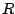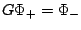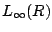# Factorization of a class of almost-periodic triangular symbols and related Riemann-Hilbert problems

Cristina Camara

The Fredholmness and invertibility of finite-interval convolution operators acting on spaces, whereis a compact interval in, is closely related to the Wiener-Hopf factorization of its matrix-valued symbol. This factorization is studied for some classes of symbolswhose entries are almost-periodic polynomials with Fourier spectrum in the group). The factorization problem is solved by calculating one solution to the Riemann-Hilbert probleminand obtaining a second linearly independent solution by means of an appropriate transformation on the space of solutions of the Riemann-Hilbert problem. Some unexpected, but interesting, results are obtained regarding the Fourier spectrum of the solutions of this class of Riemann-Hilbert problems. The Wiener-Hopf factors ofare explicitly obtained, which allow us to establish invertibility criteria and formulas for the inverse of the associated operator.